# HSSlive: Plus One & Plus Two Notes & Solutions for Kerala State Board

## BSEB Class 12 Chemistry The Solid State Textbook Solutions PDF: Download Bihar Board STD 12th Chemistry The Solid State Book AnswersBSEB Class 12 Chemistry The Solid State Textbook Solutions PDF: Download Bihar Board STD 12th Chemistry The Solid State Book Answers

BSEB Class 12th Chemistry The Solid State Textbooks Solutions and answers for students are now available in pdf format. Bihar Board Class 12th Chemistry The Solid State Book answers and solutions are one of the most important study materials for any student. The Bihar Board Class 12th Chemistry The Solid State books are published by the Bihar Board Publishers. These Bihar Board Class 12th Chemistry The Solid State textbooks are prepared by a group of expert faculty members. Students can download these BSEB STD 12th Chemistry The Solid State book solutions pdf online from this page.

## Bihar Board Class 12th Chemistry The Solid State Books Solutions

 Board BSEB Materials Textbook Solutions/Guide Format DOC/PDF Class 12th Subject Chemistry The Solid State Chapters All Provider Hsslive

## How to download Bihar Board Class 12th Chemistry The Solid State Textbook Solutions Answers PDF Online?

2. Click on the Bihar Board Class 12th Chemistry The Solid State Answers.
3. Look for your Bihar Board STD 12th Chemistry The Solid State Textbooks PDF.
4. Now download or read the Bihar Board Class 12th Chemistry The Solid State Textbook Solutions for PDF Free.

Find below the list of all BSEB Class 12th Chemistry The Solid State Textbook Solutions for PDF’s for you to download and prepare for the upcoming exams:

### Bihar Board Class 12 Chemistry The Solid State Intext Questions and Answers

Question 1. Why are solids rigid? Answer: At sufficiently low temperature, the thermal energy is low and intermolecular forces between the particles (atomic ions or molecules) of a solid bring them so close that they cling to one another and occupy fixed positions. These can still oscillate to and fro about their mean positions. Thus solids are rigid.

Question 2. Why do solids have a definite volume? Answer: For a given mass of a solid, its density has a fixed value. Hence a solid has a definite volume. [Volume = Mass density ]

Question 3. Classify the following as amorphous or crystalline solids: Polyurethane, naphthalene, benzoic acid, teflon, potassium nitrate, cellophane, polyvinyl chloride, fibre glass, copper. Answer: Crystalline solids are : Potassium nitrate, copper, benzoic acid, napthalene. Other are amorphous.

Question 4. Why is glass considered a super-cooled liquid? Answer: Because of its property to flow and its high viscosity, glass is considered a super-cooled liquid.

Question 5. Refractive index of a solid is observed to have the same value along all directions. Comment on the nature of this solid. Would it show cleavage property? Answer: The solid whose refractive index has the same value along all directions is an amorphous solid and is isotropic in nature. When such a solid is cut with a sharp edged tool, it will cut into two pieces with irregular surfaces, i.e., it does not show cleavage property.

Question 6. Classify the following solids in different categories based on the nature of intermolecular forces operating is them : Potassium sulphate, tin, benzene, urea, ammonia, water, zinc sulphide, graphite, rubidium, argon, silicon carbide. Answer:

1. Potassium sulphate: ionic solid; coulombic forces of attraction
2. Tin : metallic solid; metallic bonding
3. (solid) Benzene : molecular solid; dispersion or London forces.
4. Urea : molecular solid, dipole-dipole attractions.
5. Ammonia : molecular solid (polar) Hydrogen bonding.
6. Water (ice): molecular solid; hydrogen-bonding
7. Zinc sulphide : ionic solid, coulombic forces of attraction.
8. Graphite: Net-work or covalent, covalent bonds.
9. Rubidium : metallic solid, metallic bonds (attraction between positive metal ions called kernels and mobile electrons)
10. Argon : molecular solid, van der Waals forces.
11. Silicon Carbide (SiC) : Network or covalent solid, covalent bonds.

Question 7. Solid A is very hard electrical insulator in solid as well as in molten state and melts at extremely high temperature What type of solid is it? Answer: Network or Covalent solid.

Question 8. Ionic solids conduct electricity in molten state but not in solid state. Explain. Answer: Ions in the solid state occupy fixed positions at their lattice points in the crystal lattice. They can’t move in the solid state, but can only oscilliate to and fro about their mean position. Hence they cannot conduct electricity in the solid state. While in molten state, these ions break apart and become free to move. Thus they conduct electricity in the molten state.

Question 9. What type of solids are electrical conductors, malleable and ductile. Answer: Metallic solids are electrical conductors, malleable and ductile.

Question 10. Give the significance of a lattice point. Answer:

1. Each point in a lattice is called lattice site or lattice point.
2. Each point in a crystal lattice represents one constituent particle which may be an atom, a molecule or an ion.
3. Lattice points are joined by straight lines to bring out the geometry of the lattice.

Question 11. Name the parameters that characterise a unit cell. Answer: A unit cell is characterised by the following parameters. (i) its dimensions along the three edges a, b and c as shown. These edges may or may not be mutually perpendicular.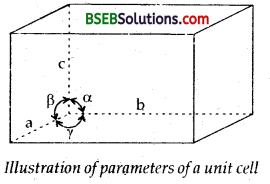(ii) angles between the edges a (between b and c), ß (between a and c) and y (between a and b). Thus a unit cell is characterised by six parameters a, b, c and α, ß, γ.

Question 12. Distinguish between (i) Hexagonal and monoclinic unit cells, (ii) Face-centered and end-centered unit cell. Answer: (i) Hexagonal unit cell is one in which three sides a = b ≠ c and three angles are α = ß = 90°, γ = 120°. Graphite arid ZnO are the examples.

Monoclinic unit cell: Though primitive like hexagonal unit cell, it is end centered. Here all the three sides are of different lengths, i.e., a ≠ b ≠ c and angles are α = γ = 90°, ß # 90°. Its example is of monoclinic sulphur.

(ii) Face centered and end-centered unit cell Face-centered unit cell contains one constituent particle present at the centre of each face, besides the ones that are at its corners. End-centered unit cell is one which contains one constituent particle at the centre of any two opposite faces besides the ones present at its comers.

Question 13. Explain how much portion of an atom located at (i) comer and (ii) body centre of a cubic unit cell is part of its neighbouring unit cell? Answer: (i) Only 1/8 th of an atom located at the comer of a cubic unit actually belongs to a particular unit cell as it (atom) is shared mutually between 8 adjacent unit cells – 4 unit cells in the same layer and four unit cells of the upper (or lower) layer.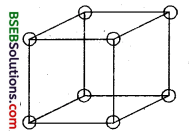A primitive cubic unit cell (open structure). Each atom at its corner is mutually shared between 8 adjacent unit cells.

(ii) An atom situated at the body centre wholly belongs to the unit cell in which it is present. It is not a part of any neighbouring unit cell.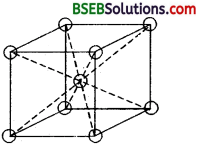A body centered cubic unit cell. The atom at the body centre wholly – belongs to the unit cell in which it is present.

Question 14. What is the two-dimensional coordination number of a molecule in square close packed layer? Answer: In this arrangement, each sphere is in contact with four of its neighbours. Thus the two dimensional coordination number is four.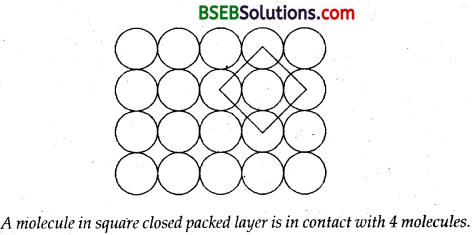Question 15. A compound forms hexagonal close-packed structure. What is the total number of voids in 0.5 mol of it? How many of these are tetrahedral voids? Answer: 0.5 mol of hexagonal closed packed structure has 0.5 mol of octahedral voids and double its no. (i.e., 1 mol of tetrahedral voids.) ∴ Total no. of voids = 3.01 x 1023 + 6.02 x 1023 = 9.03 x 1023 No. of tetrahedral voids in 0.5 mole of the unit cell 1 Mole = 6.02 x 1023 voids.

Question 16. A compound is formed by two elements M and N. The element N forms ccp and atoms of M occupy 13 rd of tetrahedral voids. What is the formula of the compound? Answer: Atoms of M occupy 13 rd of tetrahedral voids. ∴ Atoms of N will occupy 23 rd of octahedral voids as the compound forms ccp structure. Suppose thee are 90 N atoms Then total octahedral voids = 90 Hence M atoms = 23 x 90 = 60 ∴ Ratio of M : N = 60 : 90 = 2 : 3 ∴ Formula of the compound is M2N3.

Question 17. Which of the following has the highest packing efficiency (i) simple cubic, (ii) body centered cubic and (iii) hexagonal close- packed lattice. Answer: As we have seen in the text-book exercise Question 10 packing fraction or Packing efficiency is maximum in hep and ccp structures and is 74% A simple cubic has packing efficiency of only 52.4% and a body- centered cubic structure has packing efficiency of 68%. Thus the packing efficiency is maximum in the case of hexagonal close-packed lattice.

Question 18. An element with molar mass 2.7 x 10-2 kg mol-1 forms a cubic unit cell with edge length 405 pm. If its density is 2.7 x 103 kg-3, what is the nature of the cubic unit cell? Answer: Density d of the unit cell (α) = Zma3 N0. Where Z = no. of atoms present in the unit cell M = Molar mass of the substance N0 = Avogadro’s number = 6.023 x 1023 a = edge length; and a³ = Vol. of unit cellOn solving Z = 4; No. of particles in unit cell = 4 ∴ It is a cubic close-packed unit cell (ccp)

Question 19. What type of defect can arise when a solid is heated? Which physical property is affected by it and in what way? Answer: There is a perfect order in the periodic arrangement of atoms in crystal at 0 K. But with rise in temperature at 0 K, same deviations from complete order takes place. It is called defect or imperfection in crystals. Imperfections not only modify the properties but also sometimes impart new properties to the solids. On heating solids, electrons become mobile and when an electron is removed it forms an electron-deficient hole and conductivity of the solid changes. It also changes the magnetic properties associated with solids. For example conductivity of silicon is due to the mobility of these electrons.

Conductivity of metallic solids decreases on heating. Due (o anionic vacancies in ionic crystals, heating produces colour. Thus NaCl becomes yellow on heating in an atmosphere of sodium vapour. Zinc oxide which is white at room temperature becomes yellow on heating due to release of electrons ZnO → Zn2+ + 12 O2 + 2𝑒¯

There occurs a change in magnetic character of solids on healing. When a ferromagnetic on an anti-ferromagnetic or a ferrimagnetic solid is heated, it changes into paramagnetic at same temperature. With increase in temperature, there occurs a decrease in density of solid.

Question 20. What type of stoichiometric defect is shown by (i) ZnS, (ii) AgBr. Answer: (i) ZnS : It shows Frankel defect or dislocation defect. It is shown by ionic substances where there is a large difference in the size of the ions. (ii) AgBr: Ii shows both Frenkel and Schottky defect

Question 21. Explain how vacancies are introduced in an,ionic solid when a cation of higher valence is added as an impurity in it, Answer: If molten NaCl containing a little amount of SrCl2 is crystallised, some of the sites of Na+ ions are occupied by Sr2+ . Each Sr2+ replaces two sodium ions to maintain electrical neutrality. It occupies the site of one Na+ ion and the other site remains vacant. The cationic valances thus produced are equal in number to that of Sr2+ atoms.Question 22. Ionic solids, which have anionic vacancies due to metal excess defect, develop colour. Explain with the help of a suitable example. Answer: Metal excess defect due to anionic vacancies Alkali halides like NaCl, and KCl show this type of defect. When crystals of NaCl are heated in an atmosphere of sodium vapour, the sodium atoms are deposited on the surface of the crystal. The Cl– ions diffuse to the surface of the crystal and combine with Na atoms to give NaCl. This happens by loss of electron by sodium atom to form Nations. The released electrons diffuse into the crystal and occupy anionic sites.

As a result the crystal now has an excess of sodium. The anionic sites occupied by unpaired electrons are called. F-Centres (From the German word Farbe meaning colour). They impart yellow colour to the crystals of NaCl. The colour results by excitation of these electrons when they absorb energy from the visible light falling on the crystals. Similarly excess of Li makes LiCl crystals pink and excess of potassium makes KCl crystals violet.

Question 23. A group 14 element is to be converted into n-type semi-conductor by doping it with a suitable impurity. To which group should this impurity belong? Answer: The impurity should belong to group 15.

Question 24. What type of substances would make better permanent magnets, ferromagnetic or ferrimagnetic? Justify your answer. Answer: A few substances like iron, cobalt nickel, gadolinium and CrO2 are attracted very strongly by a magnetic field, such substances are called ferromagnetic. They can be permanently magnetised. In solid state, the melai ions of such ferromagnetic substances are grouped together into small regions called domains. Thus each domain acts as a tiny magnet. In an unmagnetised piece of a ferromagnetic substance the domains are randomnly oriented and their magnetic moments get cancelled. When it is placed in a magnetic field all the domains get oriented in the direction of the magnetic field and a strong magnetic effect is produced.

A ferromagnetic substance becomes a permanent magnet ever after the removal of external magnetic field. In ferrimagnetic substances like Fe3O4, the magnetic moments of the domains are aligned in parallel and anti parallel directions in unequal numbers. They are weakly attracted by magnetic field as compared to ferromagnetic substances.

### Bihar Board Class 12 Chemistry The Solid State Text Book Questions and Answers

Question 1. Define the term ‘amorphous’. Give a few examples of Amorphous solids. Answer: A solid is said to be amorphous if the constituent particles are not arranged in any regular manner. They may have only short range order. Some examples of amorphous solids are glass, plastics, rubber etc.

Question 2. What makes a glass different from a solid such as quartz? Under what conditions could quartz be converted into glass? Answer: Quartz is a crystalline form of silica (SiO2). In it SiO4 tetrahedra are connected to one another in such a way that oxygen atom of each SiO4 tetrahedron is shared with another silicon atom. If quartz or SiO2 is melted and the melt is cooled, glass is formed which is amorphous. In glass SiO4 tetrahedra are randomly joined. It is super cooled liquid.

Question 3. Classify each of the following solids as ionic, metallic, molecular, network (covalent) or amorphous.

1. Tetra phosphorous
2. Ammonium phosphate (NH4)3PO4
3. SiC
4. I2
5. P4
6. Plastic
7. Graphite
8. Brass
9. Rb
10. liBr
11. Si

1. P4O10: Molecular solid
2. Ammonium phosphate: Ionic solid
3. SiC: Covalent solid
4. I2: molecular solid
5. P4: molecular solid
6. Plastic : amorphous
7. Graphite : Covalent Solid
8. Brass : metallic solid
9. Rb : metallic solid
10. LiBr : Ionic solid
11. Si: network (covalent) solid.

Question 4. (a) What is meant by the term ‘coordination number’? (b) What is the coordination number of atoms:

• in a cubic close packed structure
• in a body centered cubic structure.

Answer: (a) The coordination number of an atom in a crystal is the number atoms which are the immediate neighbours of that atom in the crystal. In ionic crystal, coordination number of an ion in the crystal is the number of oppositely charged ions surrounding that particular ion.

(b) (i) The coordination number of atoms in a cubic close packed (cep) structure is 12, as it is touching 6 atoms in its own plane, 3 just above it and 3 just below it.

(ii) The coordination number of an atom in a body centered cubic (bcc) is 8 (as the atom in the centre of the body of the unit cell is surrounded by 8 atoms placed at the 8 comers of the cube.)

Question 5. How can you determine the atomic mass of an unknown metal if you know its density and the dimension of its unit cell? Explain. Answer: Calculation of the Atomic Mass of an Element Let the atomic mass of the element which is to be determined = M Density of the element = d g cc-1 Let the edge of the unit cell = a cm Volume of the unit cell = a³ cm³ Density of the unit cell will be the same as the density of the element. ∴ Mass of the unit cell = d x a³ g Atomic mass of the element (M) = Mass of an atom x N0where N0 = Avogadro’s number = 6.023 x 1023 Now the mass of the unit cell = No. of atoms in the unit cell x mass of each atom = Z x m . ∴ M = 6.023×1023×a3×dZ Hence Atomic mass M = N0×a3×dZ where z = 4 for fcc z = 2 for bcc z = 1 for simple cubic.

Question 6. ‘Stability of a crystal is reflected in the magnitude of its melting points’. Comment. Collect melting points of solid water, ethyl alcohol, diethyl ether and methane from a data book. What can you say about the intermolecular forces between these molecules? Answer: Melting point of solid water = 273 K M.Pt. of ethyl alcohol = 155.7 K M.Pt. of diethyl ether = 156.8 K M.Pt. of methane = 90.5 K Higher the melting point of a crystal, greater are the forces holding the constituent particles (ions/atoms) together and hence greater is its stability. The intennolecular forces in solid water (ice) and ethyl alcohol are mainly due to hydrogen bonding. Higher melting point of ice than ethyl alcohol shows that hydrogen bonding in ethyl alcohol molecules is hot as strong as in solid water molecules.

Diethyl ether is a polar molecule. The intennolecular forces present in diethyl ether actually are dipole- dipole attractions. Methane, on the other hand is a non-polar molecule. The only forces present in them are the weak van der Waal’s forces and so melting point of methane is least out of all of them and is a gas at room temperature.

Question 7. How will you distinguish between the following pairs of terms: (i) Hexagonal close packing and cubic close packing? (ii) crystal lattice and unit cell? (iii) Tetrahedral void and octahedral void? Answer: (a) Hexagonal close packing is ABAB…type whereas cubic close packing is ABCABC….type. In hexagonal close packing of spheres A, B, the central sphere’s layer B has a layer of spheres marked A above and below it so that this packing can be termed as ABAB… Here when a third layer of spheres is placed over the second layer in such a way that the spheres cover the tetrahedral voids or holes, a three-dimensional closest packing where the spheres in every third or alternate layers are vertically aligned. Both hep and ccp forms, though different, are equally efficient.Molybdenum, Magnesium and Beryllium crystallise in hep (hexagonal close packing) structure. In cubic close packing this pattern is ABCABC… Here the 3rd layer of spheres the octahedral voids. Let this layer be C. In this packing spheres in the 4th layer will be vertically aligned.Fe, Ni, Cu, Ag, Au and Al crystallise in ccp structures. Both the forms of stacking spheres occupy maximum available space.

(b) Difference between crystal lattice and unit cell – Crystal lattice or space lattice is a regular arrangement of the constituent particles (i.e. atoms, ions or molecules) of a crystal in a 3-dimensional space.

Unit Cell – The smallest three dimensional portion of a complete space lattice which when repeated over and again in different directions produces the complete space lattice is called the unit cell.(c) Tetrahedral void and Octahedral void – When we place the 2nd layer of spheres over the first layer, it can be done so only by placing the spheres on the voids/holes of the first layer. Around every sphere of the first layer, there are six voids. Thus the sphere which was touching already six spheres in one layer will also touch three more spheres in the layer above it.

The second layer contains two layers called voids, i.e., voids over the spheres of the first layer called TETRAHEDRAL VOID and the void over the void of the first layer called OCTAHEDRAL VOID. The tetrahedral void is so called because this void is surrounded by four spheres touching each other and are directed towards the comers of a tetrahedron. It has a triangular shape.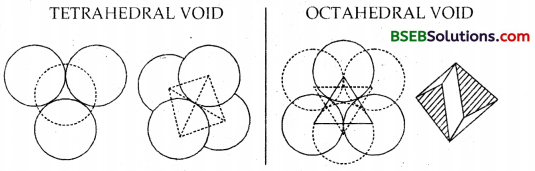Similary, the octahedral void is so called because this void is surrounded by six spheres touching each other and directed towards the corners of an octahedron. It is a combination of two triangular voids, one of the first layer and the second of the second layer with the triangle vertex upwards and the other triangle vertex downwards.

Question 8. How many lattice points are these in one unit cell of each of the following lattice? (a) face centered cubic (b) face centered tetragonal (c) body centered. Answer: (a) and (b) Lattice points in face centered cubic or face centered tetragonal – 8 (at corners) + 6 (at face centres) = 14 However particles per unit cell = 8 x 18 + 6 x 12 = 1 + 3 = 4(c) Lattice points in body centered cubic (bcc) = 8 (at corners) + 1 (at body centre) = 9 However particles per unit cell = 8 x 18 + 1 = 2Question 9. Explain : (a) The basis of similarities and differences between metallic and ionic crystals, (b) Ionic solids are hard and brittle. Answer: (a) Basis of Similarities: (i) Both ionic and metallic crystals have electrostatic forces of attraction. In ionic crystals these are between the oppositely charged ions. In metals, these are among the valence electrons which are mobile or delocalised and the fixed kernels which are positively charged. That is why both have high melting points.

(ii) In both cases the bond is non-directional.

Basis of differences: (i) In ionic crystals, the ions are not free to move. Hence they cannot conduct electricity in the solid state. They can do so only in the molten or aqueous state when they become free to move. In metals, the valence electrons being MOBILE/DELOCALIZED are free to move/flow.

Hence they can conduct electricity in the solid state. Ions carry electricity in ionic crystals when present in the molten or aqueous state and mobile electrons carry electricity in metallic crystals.

(ii) Ionic bond is a strong bond, whereas metallic bond is a weak bond.

(b) Ionic crystals are hard, because there are strong electrostatic forces of attraction among the oppositively charged ions. They are brittle because ionic bonds are non-directional.

Question 10. Calculate the efficiency of packing in case of a metal crystal for (a) simple cubic, (b) body centered cubic, (c) face centered cubic, (with the assumptions that atoms are touching each other.) Answer: (a) Calculation of efficiency in a simple cubic: Let us calculate the space occupied, i.e., packing fraction in a simple cubic unit cell. Suppose the edge length = a and radius of sphere = rAs spheres are touching each other a = 2r No. of spheres per unit cell= 18 x 8 = 1 Volume of the sphere = 43 πr³ Volume of the cube = a³ = (2r)³ = 8r³ Fraction occupied i.e., packing fraction = 43 πr³/8r³ = 0.524 % occupied = 52.4

(b) Calculation efficiency in a body centred cubic: As the sphere at the centre touches the spheres at the comers, body diagonal, AD = 4r. [The spheres at the comers are not touching each other]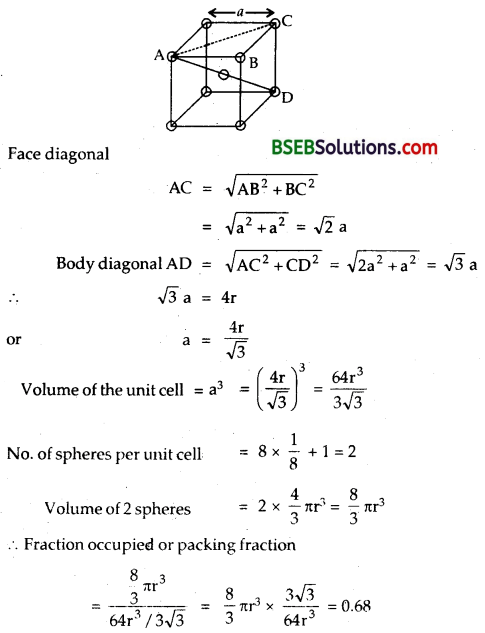Percentage occupied = 68%

(c) Calculation of efficiency in a face-centered cubic AC = 4r (as the spheres on the face are touching) But from the right angled ∆ ABC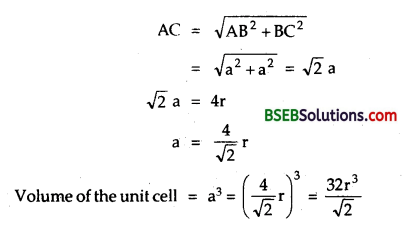Number of spheres in the unit cell = 8 x 18 + 6 x 12 = 4 Volume of 4 spheres = 4 x 43 πr³ = 163πr³ Fraction occupied, i.e., packing fraction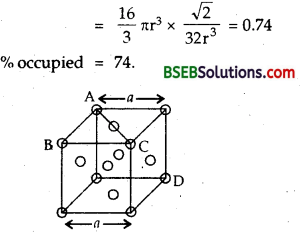Question 11. Silver crystallises in fee lattice. If edge length of the cell is 4.07 x 108 cm and density is 10.5 g cm-3, calculate the atomic mass of silver. Answer: No. of atoms in fee lattice (Z) = 8 x 18 + 6 x 12 = 4 edge length = 4.07 x 108 cm Volume = (4.07 x 108)3 = 6.74 x 10-23 cm³ Mass of the unit cell = Volume x density = 6.74 x 10-23 x 10.5 g = 7.077 x 10-22g Now Atomic Mass M = Mass of an atom (m) x Avogadro’s No.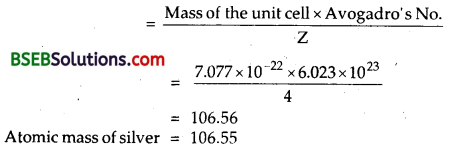Question 12. A cubic solid is made of two elements P and Q. Atoms of Q are at the comers of the cube and P at the body-center. What is the formula of the compound? What are the coordination numbers of P and Q? Answer: As atoms Q are present at the 8 comers of the cube, therefore, number of atoms of Q in the unit cell. = 18 x 8 = 1 As atoms P are present at the body centre, therefore, number of atoms of P in the unit cell = 1 Hence, the formula of the compound is PQ Coordination no. = No. of nearest atoms ∴ Coordination nos. of each of P and Q = 8

Question 13. Niobium crystallizes in body centered cubic structure. If density is 8.55 g cm-3, calculate atomic radius of niobium using its atomic mass 93 u. Answer: Atomic mass (M) = 93.0 µ Let the length of the edge of the unit cell be a cm Density of the unit cell = Mass of unit cell Volume of unit cell Mass of unit cell = No. of atoms in unit cell (Z) x Mass of each atom = Z x m∴ Atomic radius of Nb = 14.29 nm

Question 14. If the radius of the octahedral void is r and radius of the atoms in close packing is R, derive relation between r and R. Answer: Let us derive the relative between the radius of an octahedral void (r) and radius of the atoms (R) in close packing. Since all the spheres are of the same radius, ABCD is a square. ∠ABC is 90° and ∠CAB = 45°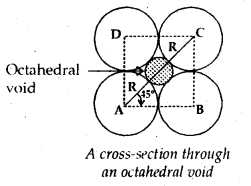∴ In the right angled triangle BCARadius of an octahedral void (r) = 0.414 R.

Question 15. Copper crystallizes into a fee lattice with edge length 3.61 x 10-8 Cm. Show that the calculated density is in agreement with its measured value of 8.92 g cm-3. Answer: Edge length of the unit cell = 3.61 x 10-8 cm Volume of the unit cell = (3.61 x 10-8)³cm³ a³ = 4.7046 x 10-23 cm³ No. of atoms of Cu in fee unit cell = 8 x 18 + 6 x 12 = 4 Mass of 1 atom of Cu = 63.506.023×1023 Mass of the unit cell = 4 x 63.50/6.023 x 1023 = 4217 x 10-22g Density of the copper crystal = 4.217×10−224.7046×10−23 = 8.97 g cm-3 which is in close agreement with the measured value of 8.92 g cm-3.

Question 16. Analysis shows that nickel oxide has formula Ni0.98O1.00. What fractions of nickel exist as Ni2+ and Ni3+ ions? Answer: Ni0.98O1.0 is a non-stoichiometric crystal. The composition of Ni ions and oxide ion = 98 :100 Out of Ni ions, let there be x Ni++ ions and (98 – x) Ni+++ ions. Since Ni0.98O1.00 is neutral. ∴ Positive charges on Ni (II) and Ni (III) must be equal io negative charges on oxide ions. x x 2 + (98 – x)3 = 100 x 2 Solving x = 94 Thus out of 98 Ni ions, There are 94 Ni++ ions and 4 are Ni+++ ions % of Ni2+ = 9498 x 100 = 96, % of NP3+ = 4%

Question 17. What is a semiconductor? Describe the two main types of semiconductors and contrast their conduction mechanism. Answer: Substances whose conductance lies between that of metals (conductors) and insulators are called semi-conductors. Two main types of semi conductors are n-type and p-type.

n-type semi – conductors Group 14 elements like Ge, Si doped with group 15 elements such as P and As are called n-type semi-conductors. The symbol ‘n’ indicating that negative charge flows in them. The group 15 elements have one excess valence electron (compared to Si or Ge) after forming the four covalent bonds normally formed by group 14 elements. The excess electrons give rise to electrical conduction.

p-type semi – conductors – A group 13 element which has only three valence electrons forms an electron-deficient bond or a hole. Such holes can move across the crystal giving rise to electrical conductivity. Thus impurity doped Si and Ge are semi-conductors whose conductivity increases with increasing temperature unlike metals whose conductivity decreases with increase in temperature.

Group 14 elements doped with group 13 elements are called p-type semi-conductors. The symbol ‘p’ is used because in an electric field, the holes move through the crystal like positive charge, i.e., in a direction opposite to the flow of electrons.

Question 18. Non-stoichiometric cuprous oxide, Cu2O can be prepared in laboratory. In this oxide, copper to oxygen ratio is slightly less than 2:1. Can you account for the fact that this substance is a p-type semiconductor? Answer: The ratio less than 2 : 1 in Cu2O shows that some cuprous ions (Cu+) have been replaced by Cupric ions (Cu 2+). To maintain electrical neutrality, every two Cu+ ions will be replaced by one Cu2+ ion thereby creating a hole. As conduction will be due to presence of these positive holes,hence it is a p-type semi-conductor.

Question 19. Ferric oxide crystallizes in a hexagonal close-packed array of oxide ions with two out of every three octahedral holes occupied by ferric ions. Derive the formula of the ferric oxide. Answer: Suppose the number of oxide ions (O2-) in the packing (hep) = 30 ∴ No. of octahedral voids = 30 As 23 rd of the octahedral voids are occupied by ferric ions, therefore, the no. of ferric ions present = 23 x 30 = 20 ∴ Ratio of Fe3+ : O2- = 20 : 30 = 2 : 3 Hence the formula of ferric oxide is Fe203.

Question 20. Classify each of the following as being either a p-type or an n-type semi-conductor. (i) Ge doped with In (ii) B doped with Si. Answer: (i) Ge is a group 14 element and In is a group 13 element. Hence an electron-deficient hole is produced and thus is a p-type semiconductor. (ii) B is group 13 element and Si is a group 14 element. Thus there will be a free electron. Hence it is an n-type semi-conductor.

Question 21. Gold (atomic radius = 0.144 nm) crystallises in a face- centered unit cell. What is the length of a side of the cell? Answer: For a face-centered cubic unit cell (fee) a = 22‾√ r = 2 x 1.414 x 0.144 nm = 0.407 nm = 4.07 x 10-8 cm. Hence, the length of the side of the unit cell = 4.07 x 10-8 cm.

Question 22. In terms of band theory, what is the difference (i) between a conductor and an insulator, (ii) between a conductor and a semi-conductor? Answer: (i) The energy gap between the valence band and conduction band in an insulator is very large whereas in a conductor, the energy gap is very small or there is overlapping between valence band and conduction band.

(ii) In a conductor, there is a very small energy gap or theie is overlapping between Valence band and conduction band, but in a semi-conductor, there is always a small energy gap between them.

Question 23. Explain the following terms with suitable examples: (i) Schottky defect (ii) Frenkel defect (iii) Interstitial (iv) F-centas. Answer: (i) Schottky Defect – If in an ionic crystal of the type A+B– equal number of cations and anions are missing from their lattice sites so that the electrical neutrality is maintained, it is called SCHOTTKY DEFECT. The Schottky defect containing one pair of holes due to missing of one cation and one anion is shown in Fig.Types of compounds exhibiting Schottky defects are those which have (i) high coordination number, (ii) small difference in the size of cations and anions. A few examples are NaCl, KCl, KBr, CsCl. As no. of ions decrease mass decreases, volume remaining constant. Thus the density of such ionic solids decreases.

(ii) Frenkel Defect – In the ionic compound of the type A+B if an ion is missing from its lattice site (causing a vacancy or a hole there) and it occupies the interstitial site, the electrical neutrality as well as the stoichiometry of the compound are maintained. This type of defect is called Frenkel defects.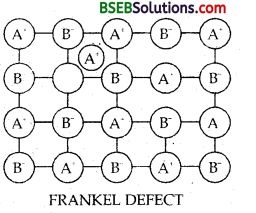Since cations are usually smaller, it is more common to find the cations occupying interstitial sites. The Frenkel defect in which one cation is missing from lattice site and is occupying the interstitial site is shown in the Fig. Types of compounds exihibiting Frenkel defect have (i) low coordination number, (ii) large difference in the size of cations and anions. It is found in silver halides due to the small size of Ag+ ion. Since no ions are missing from the crystal as a whole, density of the solid remains unchanged.

(iii) Interstitials – Atoms or ions which occupy the vacant interstitial sites are called Interstitials. The formation of interstitials depends upon the size of the atom or ion as it should be able to fit into the void. The defect that arises due to the presence of extra cations in the interstitial sites is called interstitial defect. Frenkel defect is a hybrid type of defect as it is a combination of Schottky defect and interstitial defect.

(iv) F-Centres – It arises due to non-stoich iometrie defect. The defect results in either excess of metal atoms or excess of .the non-metal atoms. Due to metal excess anionic vacancies may arise.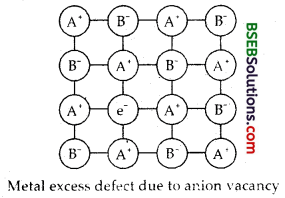A negative ion may be missing from its lattice site, leaving a hole which is occupied by an electron, thereby maintaining the electrical balance. The electrons thus trapped in the anionic vacancies are called F-Centres because they are responsible for imparting colour to the crystals (F = Farbe which is a German word for colour.]

For example when NaCl is heated in an atomosphere of Na vapour, the excess of Na atoms deposit on the surface of NaCl crystal. Cl– ions then diffuse to the surface where they combine with Na atoms which become ionised by losing electrons. These electrons diffuse back into the crystal and occupy the vacant sites created by Cl– ions. These electrons absorb some energy of the white light giving yellow colour to NaCl. Similarly excess of Li in LiCl makes it pink and excess of K in KCl makes it violet.

Question 24. Aluminium crystallizes in a cubic close-packed structure. Its metallic radius is 125 pm. (i) What is the length of the side of the unit cell? (ii) How many unit cells are there in 1.00 cm³ of aluminium? Answer: Al crystallizes in a cubic closed-packed (ccp) structure which is same as fee (face centred cubic) structure. For fcc a = 22‾√ r [r = 125 pm given] ∴ 2 x 1.414 x 125 = 354 pm ∴ length of the side of the unit cell = 354 pm Volume of one unit cell = a³ = (354 x 10-10)³ cm³ = 4.44 x 10-23 cm³ No. of unit cells in 1.00 cm³ = 4.44 x 14.44×10−23 = 2.26 x 1022

Question 25. If NaCl is doped with 10-3 mole % of SrCl2, what is the concentration of cation vacancies? Answer: Doping of NaCl with 10-3 mol % SrCl2 means that 100 moles of, NaCl are doped with 10-3 mol of SrCl2. ∴ 1 Mole of NaCl is doped with SrCl2 = 10−3100 = 10-5 mole Now each Sr2+ ion introduces one cation vacany, therefore conc. of cation vacancies = 10-5 mol/mol of NaCl = 10-5 x 6.02 x 1023 mol-1. ∴ Concentration = 6.02 x 1018 cation vacancies mol-1

Question 26. Explain the following with suitable examples: (a) ferromagnetism (b) paramagnetism (c) ferrimagnetism (d) antiferromagnetism (v) 12-16 and 13-15 group compounds. Answer: (a) Ferromagnetism – A substance which exhibits unusually in large para-magnetism and shows permanent magnetism even in absence i f the magnetic field is called Ferromagnetism substance. Examples are he, Ni, Co, CrO2, Fe3O4 and alnico (alloy of Al, Ni, Co, Fe and Cu). A ferromagnetic substance if magnetised once remains permanently magnetised. Ferromagnetism occurs due to spontaneous alignment of magnetic moments (due to unpaired electrons) in the same direction, as shown in the figure given below: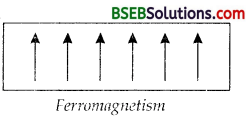(b) Paramagnetism – A substance which is attracted by a magnetic field is called Paramagnetic substance. Examples are O2, Cu2+, Fe3+. Paramagnetism is caused by the presence of permanent magnetic dipoles due to unpaired electrons in the atoms, ions or molecules. However, paramagnetic substances lose their magnetic character in the absence of magnetic field.

(c) Ferrimagnetism – A substance which exhibits fairly good paramagnetic character is called Ferrimagnetic substance. Examples are Fe3O4, ferrites having the formula M2+Fe2O4 (where M2+ = Mg2+ Cu2+ or Zn2+)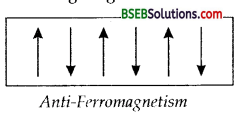In ferrimagnetic substances, the alignment of magnetic moments in opposite directions are not equal. As a result, the substance has net magnetic moment. Fe3O4 (a ferrimagnetic substance) on heating to 850 K becomes paramagnetic because at this tempearture, there is greater alignment of spins in one direction.

(d) Anti-Ferromagnetism – Substances which are expected to possess paramagnetism or ferromagnetism on the basis of unpaired electrons, but actually they posses zero net magnetic moment are called anti-ferromagnetic substances, e.g., MnO. Antiferromagnetism is due to the presence of equal number of magnetic moments in the opposite directions as shown in the figure given below.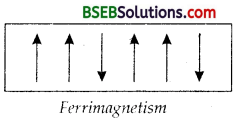(e) 12-16 and 13-15 Group Compounds – The solid binary compounds prepared by combining elements of group 13 and 15 or group 12 and 16 are classified as :

• 12-16 Compounds: ZnS, CdS, CdSe, Hg Te have sufficient ionic nature.
• 13-15 Compounds: AlP, GaAs, InSb have large covalent nature Both (i) and (ii) compounds have interesting electrical and optical properties. The compounds are semi-conductors.

### Bihar Board Class 12 Chemistry The Solid State Additional Important Questions and Answers

Question 1. Which out of and CsCl, has larger coordination number? Answer: CsCl = 8; NaCl = CsCl has larger coordination number.

Question 2. Name one solid which shows both Frenkel and Schottky defects. Answer: AgBr.

Question 3. What happens to the structure of CsCl when it is heated to about 760 K? Answer: CsCl structure is transformed to NaCl structure.

Question 4. Arrange simple cubic, body centred cubic, face-centred cubic and hexagonal close packing in the increasing order of the fraction of occupied space. Answer: Simple cubic < bcc < fee.

Question 5. What is the effect of Schottky defects on the density of the crystal. Answer: The overall density of the crystal decreases.

Question 6. Why is Frenkel defect not found in pure metal halides? Answer: Because in metal (alkali) halides, ions cannot get into interstitial sites due to their large sizes.

Question 7. In a close packing of N spheres, how many (i) tetrahedral and (ii) octahedral sites are present? Answer:

1. Tetrahedral = 2N
2. Octahedral = N.

Question 8. What is photovoltaic cell? Answer: Amorphous silicon acts as a typical photovoltaic cell in which as a result of sunshine (sunlight) electricity is produced.

Question 9. How does temperature influence the conductivity of a cell? Answer: Its conductivity increases with increase in temperature.

Question 10. What is the difference in semi-conductor obtained by doping silicon with As or with Ga? Answer: Si produced by doping with As is n-type semi-conductor in which flow of current is due to electrons while silicon doped with Ga is p-type semi-conductor in-which flow of current is due to positive holes.

Question 11. Give three examples of amorphous solids. Answer: Glass, rubber and plastics.

Question 12. What is Schottky defect? Answer: When equal number of cations and anions are missing from their lattice sites.

Question 13. What are interstitials in a crystals? Answer: Atoms or ions that fill the normal vacant interstitial voids in a crystal are called interstitials.

Question 14. What is the effect of presence of Frenkel defects on the density of a crystal? Answer: The density of the crystal does not change.

Question 15. How many atoms are present in the unit cell of (a) Simple cubic lattice, (b) BCC, (c) FCC? Answer: (a) 1 (b) 2 and (c) 4.

Question 16. How can you convert NaCl structure into CsCl structure and vice-versa? Answer: NaCl structure can be converted into CsCl structure by application of pressure, while reverse can be done by heating CsCl to 760 K.

Question 17. What is the coordination number of NA+ and Cl- ions in NaCl structure? Answer: Six.

Question 18. At what temperature ferrimagnetic substance changes to paramagnetic? Answer: 850 K.

Question 19. In a crystal of ZnS, Zinc occupies tetrahedral voids. What is the coordination nu rnber of Zinc? Answer: 4.

Question 20. What is meant by superconductivity? Answer: At low temperature when a substance offers no resistance to the flow of electricity, the phenomenon is known as superconductivity.

Question 21. What is energy gap in band theory? Compare its size in conductors, semi-conductor and insulators? Answer: Energy gap in band theory means the gap of energy between valence band and conduction band. The gap is in order conductor < semi-conductor < insulator.

Question 22. What is F-centre? Answer: The site where electron is enlrupped in the anion vacancy is called F-centre.

Question 23. What is the maximum possible coordination number of an atom in an-hep crystal structure of an element? Answer: 12.

Question 24. What happens when ferrimagnetic Fe304 is heated to 850 K and Why? Answer: Ferrimagnetic Fe3O4 on heating to 850 K becomes paramagnetic. This is due to greater alignment of spins in one direction on heating.

Question 25. In CaF2 crystal. Ca2+ ions are present in fee arrangement. Calculate the number of F– ions in the unit cell. Answer: No. of Ca2+ ions per unit cell = 8 x 18 + 6 x 12 = 4. Hence, no. of F– ions per unit cell = 2 x 4 = 8

Question 26. What is the coordination number of an octahedral void? Answer: Six.

Question 27. What type of crystal defect is produced when NaCl is doped with MgCl2? Answer: It is called impurity defect. A cation vacancy is produced. A substitutional solid solution is formed (because 2Na+ ions are replaced by one Mg2+ ions in the lattice).

Question 28. Mention one property which is caused due to the presence of F-centre in a solid. Answer: F-centre is responsible for the colour and paramagnetic behaviour.

Question 29. At what temperature range most of the metals became superconductors? Answer: 2K – 5K.

Question 30. How can a material be made amorphous? Answer: By melting the material and then cooling it suddenly.

Question 1. Calculate the approximate number of unit cells present in 1 g of gold. Given that gold crystallises in the fee lattice. [Atomic mass of gold = 197 u] Answer: 1 mole of gold = 197 g = 6.02 x 1023 atoms as fee lattice contains 4 atoms ∴ No. of unit cells present = 6.02×1023197×4 = 7.64.x 1020

Question 2. Calculate the numbers of atoms in a cubic based unit cell having one atom on each comer and two atoms on each body diagonal. Answer: There are 4 body diagonals. ∴ No. of atoms present in the body = 4 x 2 = 8 No. of atoms at corners of the cube = 8 x 18 = 1 Hence total no. of atoms in the unit cell = 8 + 1 = 9

Question 3. If three atoms P, Q and R crystallise in a cubic solid lattice with P atoms at the corners, Q atoms at the cube centre and R atoms at the centre of the faces of the cube, write the formula of compound. Answer: As P atoms are at corners, total no. of atoms in the unit cell = 8 x 18 = 1 As Q is in th6 centre of the cube, No. of Q atoms/ unit cell = 1 x 1 = P As R atoms are at the face centres ∴ No. of R atoms/unit cell = 6 x 18 = 3 Hence the formula is PQR3.

Question 4. A compound formed by elements X and Y crystallises in the cubic structure where Y atoms are at the corner of the cube and X atoms are at the alternate faces. What is the formula of the compound? Answer: No. of Y atoms in the cube = 8 x 18 = 1 There can be only two X atoms on alternate faces ∴ No. of X atoms/unit cell = 2 x 12 = 1 ∴ Formula of the compound is XY.

Question 5. A solid AB has NaCl structure. If the radius of cation A+ is 170 pm, calculate the maximum possible radius of anion B–. Answer: The formula r+ = 0.414 x r– [as AB has NaCl octahedral structure] gives maximum possible radius of anion for a given cation for close packing = 𝑟+0.414 = 1700.414 = 410.6 pm.

Question 6. A solid is made up of two elements P and Question Atoms Q are in ccp arrangement while atoms P occupy are the tetrahedral sites. What is the formula of the compound? Answer: Suppose no. of Q atoms = n Then no. of tetrahedral sites = 2n ∴ No. of atoms of P = 2n ∴ Ratio P : Q = 2n : n = 2 : 1 Hence formula is P2Q.

Question 7. Br– ions form a close packed structure. If the radius of Br ion is 195 pm, calculate the radius of the cation that just fits into the tetrahedral hole. Can a cation A+ having a radius of 82 pm be slipped into the octahedral hole of the crystal A+Br–? Answer: (i) Radius of the cation just fitting into the tetrahedral hole = radius of the tetrahedral hole = 0.225 x rBr– = 43.875 pm

(ii) For the cation A+ radius = 82 pm radius ratio = 𝑟+𝑟− = 82195 = 0.4205 As it lies in the range 0.414 – 0.732, hence the cation Ah can be slipped into the octahedral hole of the crystal A+Br–.

Question 8. Do all metals possess a close-packed structure? Name the different structures exhibited and give their packing fractions. Answer: No, metals with hep and ccp arrangement have close-packed structure with packing fraction = 0.74, where as metals with bcc do not have close packed structure as packing fraction is 0.68.

Question 9. Zinc oxide is white, but it turns yellow on heating. Explain. Answer: When ZnO is heated, it loses oxygen. ZnO → Zn2++ 12 O2 + 2e– Zn2+ ions are entrapped in the interstitial voids and electrons in the neighbouring interstitial voids to maintain electrical neutrality. This gives rise to metal excess defect. Due to presence of electrons in the interstitial voids, the colour is yellow.

Question 10. Why the window glass of the old buildings looks milky? Answer: It is due to heating during day and cooling at night, i.e., over a number of years,.glass acquires same crystalline character.

Question 11. Why is the window glass of the old buildings thick at the bottom? Answer: Glass is not a true solid, but a supercooled liquid of high viscosity (called pseudo-solid). It has the property to flow.

Question 12. The electrical conductivity of a metal decreases with rise in temperature while that of a semi conductor increases. Explain. Answer: In case of metals, with increase in temperature, the kernels start vibrating and thus offer resistance to the flow of mobile electrons. So conductivity decreases. In semi-conductors, the conductivity is due to the presence of impurities and defects. As, the no. of defects (such as holes) increase with increase in temperature, conductivity increases.

Question 13. Why does zinc oxide exhibit enhanced electrical conductivity on heating? Answer: On heating zinc oxide loses oxygen as follows :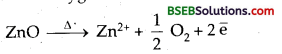The Zn2+ ions are trapped into the interstitial sites while the electrons are also entrapped in the neighbouring interstitial sites. These trapped electrons enhance the electrical conductivity of ZnO.

Question 14. What is the difference between anti-ferromagnetic and ferrimagnetic substance? What is the cause of this difference? Answer: Anti-ferromagnetic substances possess zero net magnetic moment but ferromagnetic possess small net magnetic moment but not zero. This is because the former contain equal number of electrons with opposite spin, but the latter have unequal number.

Question 15. Why are the defects of the crystalline solids called thermodynamic defects? Answer: There is perfect arrangement of the constituent particles only at O K. As the temperature increases, the chances of lattice being unoccupied by ion increases. As the no. of defects increase with temp, the defects are called thermodynamic defects.

Question 1. (a) Define crystal lattice and unit cell. Describe their characteristics. (b) What are the characteristics of solids? What are amorphous and crystalline solids. Answer: (a) Crystal Lattice – A regular three dimensional arrangement of points (particles-atoms, ions or molecules) in space is called a crystal lattice. The following are the characteristics of a crystal lattice :

• Each point in a lattice is called a lattice point or lattice site.
• Each point in a crystal lattice represents one constituent particle which may be an atom, a molecule or an ion.
• Lattice points are joined by straight lines to bring out the geometry of the lattice.

Unit Cell – It is the smallest portion of a crystal lattice which, when repeated in different directions, generates the entire lattice.

• A unit cell is characterised by its dimensions along the three edges a, b and c. These edges may or may not be mutually perpendicular.
• Angles between the edges α (between b and c), ß (between a and c) and γ (between a and b). Thus a unit cell s characterised by six parameters a, b and c and α, ß, γ.

(b) The following are the characteristics of solid state:

• They have definite mass, volume and shape.
• Intermolecular distances are short.
• Intermolecular forces are strong.
• Their constituents particles (atoms, molecules or ions) have fixed positions and can oscilliate about their mean positions only.
• They are incompressible and rigid.

Amorphous and Crystalline Solids – Solids can be classified as crystalline or amorphous on the basis of nature of order present in the arrangement of their constituent particles.

A Crystalline solid usually consists of a large no. of small crystals each of them having a definite characteristic geometrical shape. In a crystal the arrangement of constituent particles (atoms, molecules or ions) is ordered. It has long range order. NaCl and quartz are typical examples of crystalline solids.

An amorphous solid consists of particles of irregular shape. The I arrangement of constituent particles (atoms molecules or ions) in such a solid has only short-range order. In such an arrangement, a regular and periodically repeating is observed over short distances only, such portions are scattered and in between the arrangement is disordered. Quartz glass is an example of amorphous solid, glass, rubber and plastic are other examples.

Multiple Choice Questions

Question 1. Which of the following transition metal oxides is paramagnetic? (a) TiO (b) VO (c) Cu2O (d) Mn2O3 Answer: (b) VO

Question 2. Which of the following transition metal oxides is not an insulator? (a) MnO (b) NiO (c) VO (d) Mn2O3 Answer: (c) VO

Question 3. Coordination number in ccp and hep arrangements of metal atoms are respectively. (a) 6, 6 (b) 12,6 (c) 8, 6 (d) 12,12 Answer: (d) 12,12

Question 4. An example of body centered cube is _______. (a) Sodium (b) Magnesium (c) Zinc (d) Copper Answer: (a) Sodium

Question 5. Fe3O4 is ferrimagnetic at room temperature but at 850 K it becomes _______. (a) Diamagnetic (b) Ferromagnetic (c) Non-magnetic (d) Paramagnetic Answer: (d) Paramagnetic

Question 6. Which of the following is not an example of 13-15 compounds. (a) InSb (b) GaAs (c) CdSe (d) Alp Answer: (c) CdSe

Question 7. For tetrahedral coordination, the radius ration (r+/r– ) should be (a) 0.155-0.225 (b) 0.225-0.414 (c) 0.414-0.732 (d) 0.732-1 Answer: (b) 0.225-0.414

Question 8. A compound formed by elements A and B crystallises in the cubic structure where A atoms are at comers of a cube and B atoms are at face centres. The formula of the compound is _______. (CBSE PMT 2000, AIEEE 2005) (a) AB3 (b) A2B3 (c) AB2 (d) A2B3 Answer: (a) AB3

Question 9. How many space lattices are possible in a crystal? (a) 23 (b) 7 (c) 230 (d) 14 Answer: (d) 14

Question 10. The number of atoms in bcc arrangement is _______. (a) 1 (b) 2 (c) 4 (d) 6 Answer: (b) 2

Question 11. In face-centered cubic unit cell, edge length is _______. (DPMT 2005) (a) 43√ (b) 42√ (c) 2r (d) 3√2r Answer: (b) 42√

Question 12. Metallic lustre is explained by _______. (a) diffusion of metal ions (b) oscillation of loose electrons (c) excitation of free protons (d) existence of bcc lattice. Answer: (b) oscillation of loose electrons

Question 13. In a face-centered cubic lattice, unit cell is shared equally by how many unit cells? (CBSE P.M.T. 2005) (a) 4 (b) 2 (c) 6 (d) 8 Answer: (c) 6

Question 14. The percentage of iron present as Fe (III) in Fe0.93 O1.0 is _______. (a) 17.7% (b) 7.84% (c) 11.5% (d) 9.6% Answer: (c) 11.5%

Question 15. Which of the following fee structure contains cations in alternate tetrahedral voids? (IIT 2005) (a) NaCl (b) ZnS (c) Na2 (d) CaF2 Answer: (b) ZnS

Question 16. The numbers of tetrahedral voids in the unit cell of a face centered cubic lattice of similar atoms is (Kerala MEE 2004) (a) 4 (b) 6 (c) 8 (d) 10 (e) 12 Answer: (c) 8

Question 17. The hardest substance among the following is : (Kerala MEE 2004) (a) Be2C (b) Graphite (c) Titanium (d) SiC (e) B4C Answer: (d) SiC

Question 18. How many unit cells are present in a cube-shaped ideal crystal of NaCl of mass 1.00 g? (Atomic mass of Na = 213, Cl = 35.5) (AIEEE 2003) (a) 5.14 x 1021 (b) 1.28 x 1021 (c) 1.71 x 1021 (d) 2.57 x 1021 Answer: (d) 2.57 x 1021

Question 19. What is the coordination number of Cl– in NaCl crystals _______. (Orissa J.E.E. 2003) (a) 8 (b) 6 (c) 4 (d) 3 Answer: (b) 6

Question 20. The pyknometric density of sodium chloride crystal is 2.165 x 103 kg m-3, while its X-ray density is 2.178 x 103 kg m-3. The fraction of the unoccupied sites in sodium chloride crystal is _______. (CBSE P.M.T. 2003) (a) 5.96 (b) 15.96 x 10-2 (c) 5.96 x 10-1 (d) 5.96 x 10-3 Answer: (d) 5.96 x 10-3

Question 21. If Z is the number of atoms in the unit cell that represents the closest packing sequence….ABC ABC….the number of tetrahedral voids in the unit cell is equal to _______. (AIIMS…2005) (a) Z (b) 2Z (c) Z/2 (d) Z/4 Answer: (b) 2Z

Question 22. Which has no rotation of symmetry? (Orissa JEE 04) (a) Hexagonal (b) Orthorhombic (c) Cubic (d) Triclinic Answer: (d) Triclinic

Question 23. If the distance between Na+ and Cl– ions in sodium chloride crystal is X pm the length of the edge of the unit cell is _______. (Karnataka CF.T 2004) (a) 4X pm (b) X/4 pm (c) X/2 pm (d) 2 x pm Answer: (d) 2 x pm

Question 24. Which of the following metal oxides is antiferromagnetic in nature? (MP PET 2002) (a) MnO2 (b) TiO2 (c) VO2 (d) CrO2 Answer: (d) CrO2

Question 25. The interionic distance for cesium chloride crystal will be _______. (MP PET 2002) (a) a (b) a/2 (c) 3‾√ a/2 (d) 2a/3‾√ Answer: (c) 3‾√ a/2

Question 26. The liquified metal expanding on solidification is (AIIMS 2004) (a) Ga (b) Al (c) Zn (d) Cu Answer: (a) Ga

Question 27. The number of unit cells in 58.5 g of NaCl is nearly _______. (MPPMT2000) (a) 6 x 1020 (b) 3 x 1022 (c) 1.5 x 1023 (d) 0.5 x 1024 [Hint: 58.5 g NaCl = 1 mole = 6.02 x 1023 NaCl units. One unit cell contains 4 NaCl units. Hence no. of unit cells present in present in 58.5 g NaCl = 6.02 x 1023/4 = 1.5 x 1023] Answer: (d) 0.5 x 1024

Question 28. The number of octahedral sites per sphere in fee structure is _______. (MP PMT 2000) (a) 8 (b) 4 (c) 2 (d) 1 Answer: (d) 1

Question 29. The crystal system of a compound with unit cell dimensions a = 0.387, b = 0.387 and 0.504 nm and α = ß = 90° and γ = 120° is _______. (AIIMS 2004) (a) Cubic (b) Hexagonal (c) Orthorhombic (d) rhombohedral Answer: (b) Hexagonal

Question 30. Which type of crystal defect is indicated in the diagram below? (AIEEE O3)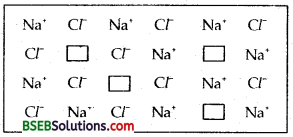(a) Frenkel defect (b) Schottky defect (c) Interstitial defect (d) Frenkel and Schottky defects. Answer: (b) Schottky defect

## Bihar Board Class 12th Chemistry The Solid State Textbooks for Exam Preparations

Bihar Board Class 12th Chemistry The Solid State Textbook Solutions can be of great help in your Bihar Board Class 12th Chemistry The Solid State exam preparation. The BSEB STD 12th Chemistry The Solid State Textbooks study material, used with the English medium textbooks, can help you complete the entire Class 12th Chemistry The Solid State Books State Board syllabus with maximum efficiency.

## FAQs Regarding Bihar Board Class 12th Chemistry The Solid State Textbook Solutions

#### Can we get a Bihar Board Book PDF for all Classes?

Yes you can get Bihar Board Text Book PDF for all classes using the links provided in the above article.

## Important Terms

Bihar Board Class 12th Chemistry The Solid State, BSEB Class 12th Chemistry The Solid State Textbooks, Bihar Board Class 12th Chemistry The Solid State, Bihar Board Class 12th Chemistry The Solid State Textbook solutions, BSEB Class 12th Chemistry The Solid State Textbooks Solutions, Bihar Board STD 12th Chemistry The Solid State, BSEB STD 12th Chemistry The Solid State Textbooks, Bihar Board STD 12th Chemistry The Solid State, Bihar Board STD 12th Chemistry The Solid State Textbook solutions, BSEB STD 12th Chemistry The Solid State Textbooks Solutions,
Share: